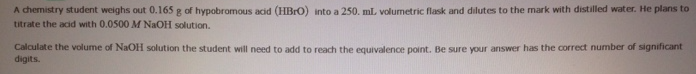# Problem: A chemistry student weighs out 0.165 g of hypobromous acid (HBrO) into a 250 mL volumetric flask and dilutes to the mark with distilled water. He plans to titrate the acid with 0.0500 M NaOH solution Calculate the volume of NaOH solution the student will need to add to reach the equivalence pant. Be sure your answer has the correct number of significant digits.

###### FREE Expert Solution
80% (173 ratings)###### Problem Details

A chemistry student weighs out 0.165 g of hypobromous acid (HBrO) into a 250 mL volumetric flask and dilutes to the mark with distilled water. He plans to titrate the acid with 0.0500 M NaOH solution Calculate the volume of NaOH solution the student will need to add to reach the equivalence pant. Be sure your answer has the correct number of significant digits.